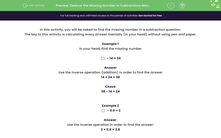# Use Mental Methods to Solve Subtraction Problems

In this worksheet, students will be asked to use efficient mental methods to subtract mentally. They will use the inverse operation to calculate a missing number.Key stage:  KS 2

Curriculum topic:   Number: Addition and Subtraction

Popular topics:   Subtraction worksheets

Difficulty level:#### Worksheet Overview

In this activity, you will be asked to find the missing number in a subtraction question.

The key to this activity is calculating every answer mentally (in your head) without using pen and paper.

Example 1- 14 = 24

14 + 24 = 38

Check

38 - 14 = 24

Example 2- 0.6 = 2

Use the inverse operation in order to find the answer:

2 + 0.6 = 2.6

Check

2.6 - 0.6 = 2

Example 3

34 -= 11

Use the numbers you have been given to complete a subtraction sum in order to find the missing number:

34 - 11 = 23

Check

34 - 23 = 11

This method will make more sense when you attempt to answer some questions.

### What is EdPlace?

We're your National Curriculum aligned online education content provider helping each child succeed in English, maths and science from year 1 to GCSE. With an EdPlace account you’ll be able to track and measure progress, helping each child achieve their best. We build confidence and attainment by personalising each child’s learning at a level that suits them.

Get started#### Popular Maths topics

••••